# 图形界面 X: 渲染表格的新功能 (集成编译 9)

• 概论

• 格式化斑马样式

• 选择和弃选表格行

• 列标题

• 相对于列宽调整字符串长度

• 事件处理

• 结论

### 概论

• 图形界面 VII: 表格控件 (第 1 章)

• 图形界面 X: 函数库的轻松快捷更新 (集成编译 3)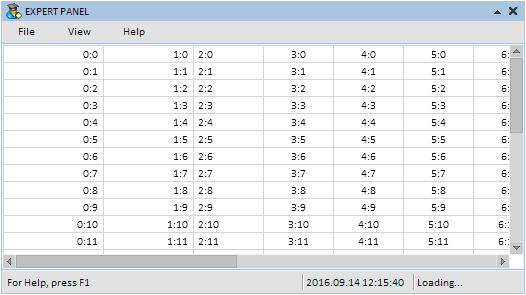• 格式化斑马样式

• 选择表格行, 再次单击时弃选

• 添加了列标题, 可在鼠标悬浮和点击时改变颜色

• 当单元格空间不足时, 则依照文本自动调整列宽

• 能够通过拖动其边框来改变每列的标题宽度

### 格式化斑马样式

```//++//| 创建渲染表格的类                                                    |//++class CCanvasTable : public CElement
{private:
//- 格式化斑马样式模式   color             m_is_zebra_format_rows;
//-public:
//- 格式化斑马样式   void              IsZebraFormatRows(const color clr)   { m_is_zebra_format_rows=clr;      }
};```

```class CCanvasTable : public CElement
{public:
//- 绘制表格行的背景   void              DrawRows(void);
};//++//| 绘制表格行的背景                                                    |//++void CCanvasTable::DrawRows(void)
{//- 如果禁用格式化斑马样式模式   if(m_is_zebra_format_rows==clrNONE)
{
//- 用一种颜色填充画板      m_table.Erase(::ColorToARGB(m_cell_color));      return;
}//- 标题坐标   int x1=0,x2=m_table_x_size;
int y1=0,y2=0;//- 格式化斑马样式   for(int r=0; r<m_rows_total; r++)
{
//- 计算坐标      y1=(r*m_cell_y_size)-r;
y2=y1+m_cell_y_size;
//- 行颜色      uint clr=::ColorToARGB((r%2!=0)? m_is_zebra_format_rows : m_cell_color);
//- 绘制行背景      m_table.FillRectangle(x1,y1,x2,y2,clr);     }
}```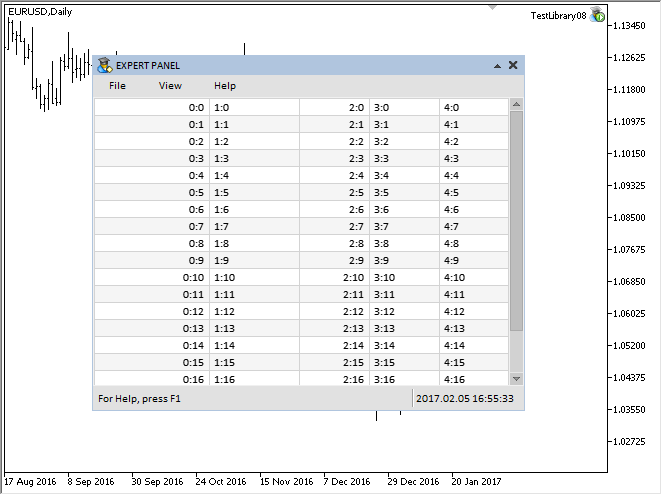### 选择和弃选表格行

• 所选行的背景和文本的颜色

• 索引和文本

```class CCanvasTable : public CElement
{private:
//- 颜色用于 (1) 背景 (2) 所选行文本   color             m_selected_row_color;
color             m_selected_row_text_color;
//- (1) 索引 (2) 所选行文本   int               m_selected_item;
string            m_selected_item_text;
//-public:
//- 返回 (1) 索引 (2) 表格中所选行文本   int               SelectedItem(void)             const { return(m_selected_item);         }
string            SelectedItemText(void)         const { return(m_selected_item_text);    }
//-private:
//- 绘制表格行的背景   void              DrawRows(void);
};```

```class CCanvasTable : public CElement
{private:
//- 可选行模式   bool              m_selectable_row;
//-public:
//- 行选择   void              SelectableRow(const bool flag)       { m_selectable_row=flag;           }
};```

```class CCanvasTable : public CElement
{private:
//- 绘制所选行   void              DrawSelectedRow(void);
};//++//| 绘制所选行                                                         |//++void CCanvasTable::DrawSelectedRow(void)
{//- 设置初始坐标以便检查条件   int y_offset=(m_selected_item*m_cell_y_size)-m_selected_item;//- 坐标   int x1=0,x2=0,y1=0,y2=0;//-   x1=0;
y1=y_offset;
x2=m_table_x_size;
y2=y_offset+m_cell_y_size-1;//- 绘制填充矩形   m_table.FillRectangle(x1,y1,x2,y2,::ColorToARGB(m_selected_row_color));  }```

```class CCanvasTable : public CElement
{private:
//- 返回单元格文本的颜色   uint              TextColor(const int row_index);
};//++//| 返回单元格文本的颜色                                                 |//++uint CCanvasTable::TextColor(const int row_index)
{
uint clr=::ColorToARGB((row_index==m_selected_item)? m_selected_row_text_color : m_cell_text_color);//- 返回标题颜色   return(clr);
}```

• 行选择模式被禁用;

• 滚动条已激活;

• 未在表格上点击。

• ON_CLICK_LIST_ITEM 事件的标识符

• 控件的标识符

• 所选行的索引

• 所选行的文本。

```class CCanvasTable : public CElement
{private:
//- 在元素上点按处理   bool              OnClickTable(const string clicked_object);
};//++//| 点击控件处理                                                       |//++bool CCanvasTable::OnClickTable(const string clicked_object)
{//- 如果禁用选择行模式, 离开   if(!m_selectable_row)
return(false);//- 如果滚动条激活, 离开   if(m_scrollv.ScrollState() || m_scrollh.ScrollState())
return(false);//- 如果对象名不同, 离开   if(m_table.Name()!=clicked_object)
return(false);//- 获取 X 和 Y 轴的偏移量   int xoffset=(int)m_table.GetInteger(OBJPROP_XOFFSET);   int yoffset=(int)m_table.GetInteger(OBJPROP_YOFFSET);//- 确定鼠标光标下方的文本编辑框坐标   int y=m_mouse.Y()-m_table.Y()+yoffset;//- 确定点击的行   for(int r=0; r<m_rows_total; r++)
{      //- 设置初始坐标以便检查条件      int y_offset=(r*m_cell_y_size)-r;
//- 沿 Y 轴检查状态      bool y_pos_check=(y>=y_offset && y<y_offset+m_cell_y_size);
//- 如果点击并非此行, 则转至下一个      if(!y_pos_check)
continue;
//- 如果点击已选行, 取消选择      if(r==m_selected_item)
{
m_selected_item      =WRONG_VALUE;
m_selected_item_text ="";
break;
}
//- 保存行索引       m_selected_item      =r;
m_selected_item_text =m_vcolumns.m_vrows[r];
break;
}//- 表格重绘   DrawTable();//- 发送有关消息   ::EventChartCustom(m_chart_id,ON_CLICK_LIST_ITEM,CElementBase::Id(),m_selected_item,m_selected_item_text);
return(true);
}```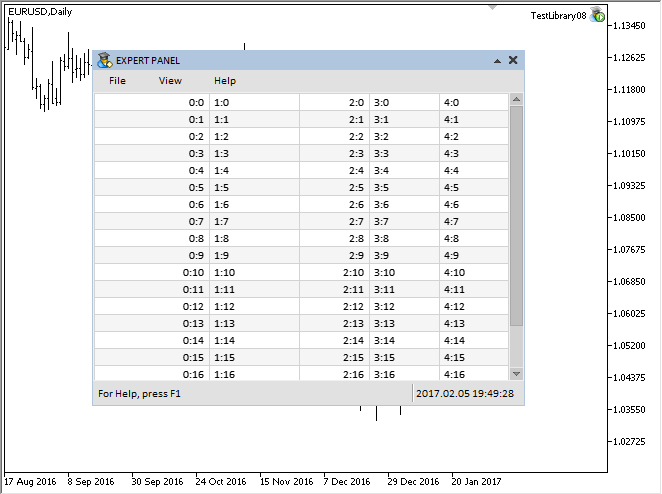### 列标题

```class CCanvasTable : public CElement
{private:

• 表头的显示模式。

• 标题的大小 (高度)。

• 不同状态下的标题背景颜色。

• 标题文本颜色。

```class CCanvasTable : public CElement
{private:
//- 不同状态下的标题 (背景) 颜色   color             m_headers_color;
//-public:
//- (1) 标题显示模式, (2) 标题高度   void              ShowHeaders(const bool flag)         { m_show_headers=flag;             }
//- (1) 背景 (2) 标题文本颜色   void              HeadersColor(const color clr)        { m_headers_color=clr;             }
};```

```class CCanvasTable : public CElement
{private:
//- 设置指定标题的文本   void              SetHeaderText(const int column_index,const string value);  };//++//| 在指定索引处填充标题数组                                              |//++void CCanvasTable::SetHeaderText(const uint column_index,const string value)  {//- 检查列超界   uint csize=::ArraySize(m_vcolumns);
if(csize<1 || column_index>=csize)
}```

```class CCanvasTable : public CElement
{private:
//- 返回指定列中的文本对齐模式   uint              TextAlign(const int column_index,const uint anchor);
};//++//| 返回指定列中的文本对齐模式                                            |//++uint CCanvasTable::TextAlign(const int column_index,const uint anchor)
{
uint text_align=0;//- 当前列的文本对齐   switch(m_vcolumns[column_index].m_text_align)
{
case ALIGN_CENTER :
text_align=TA_CENTER|anchor;
break;
case ALIGN_RIGHT :
text_align=TA_RIGHT|anchor;
break;
case ALIGN_LEFT :
text_align=TA_LEFT|anchor;
break;
}//- 返回对齐类型   return(text_align);
}```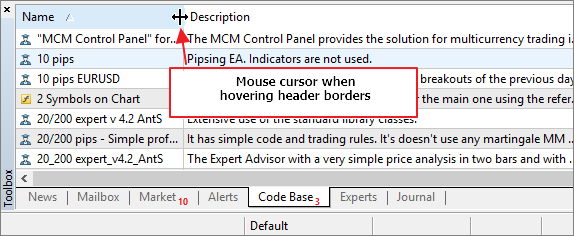```//++//| 指针类型的枚举                                                      |//++enum ENUM_MOUSE_POINTER
{
MP_CUSTOM            =0,
MP_X_RESIZE          =1,
MP_Y_RESIZE          =2,
MP_XY1_RESIZE        =3,
MP_XY2_RESIZE        =4,   MP_X_RESIZE_RELATIVE =5,   MP_Y_RESIZE_RELATIVE =6,
MP_X_SCROLL          =7,
MP_Y_SCROLL          =8,
MP_TEXT_SELECT       =9  };```

```//++//|                                                      Pointer.mqh |//|                                 版权所有 2015, MetaQuotes 软件公司  |//|                                              https://www.mql5.com |//++//- 资源#resource "\\Images\\EasyAndFastGUI\\Controls\\pointer_x_rs_rel.bmp"#resource "\\Images\\EasyAndFastGUI\\Controls\\pointer_y_rs_rel.bmp"//++//| 创建鼠标光标的类                                                    |//++class CPointer : public CElement
{private:
//- 设置鼠标光标的图像   void              SetPointerBmp(void);
};//++//| 基于光标类型设置光标图标                                              |//++void CPointer::SetPointerBmp(void)
{
switch(m_type)
{
...      case MP_X_RESIZE_RELATIVE :         m_file_on  ="Images\\EasyAndFastGUI\\Controls\\pointer_x_rs_rel.bmp";
m_file_off ="Images\\EasyAndFastGUI\\Controls\\pointer_x_rs_rel.bmp";
break;
case MP_Y_RESIZE_RELATIVE :
m_file_on  ="Images\\EasyAndFastGUI\\Controls\\pointer_y_rs_rel.bmp";
m_file_off ="Images\\EasyAndFastGUI\\Controls\\pointer_y_rs_rel.bmp";
break;
...
}//- 如果指定为自定义类型 (MP_CUSTOM)   if(m_file_on=="" || m_file_off=="")
::Print(__FUNCTION__," > 必须为光标设置两个图像");
}```

• 确定拖动标题边框的时刻

• 为了确定鼠标光标从一个标题区域移动到另一个标题区域的时刻。这需要节省资源, 因此只有当相邻区域的边界交错时才会重新绘制标题。

```class CCanvasTable : public CElement
{private:
//- 确定鼠标光标从一个标题转换到另一个标题的时刻   int               m_prev_header_index_focus;   //- 拖动标题边框以改变列宽的状态   int               m_column_resize_control;  };```

```class CCanvasTable : public CElement
{private:
{
else     {
//- 如果鼠标左键按下且未处于列宽改变进程      bool condition=(m_mouse.LeftButtonState() && m_column_resize_control==WRONG_VALUE);
}//- 返回标题颜色   return(::ColorToARGB(clr));
}```

CCanvasTable::DrawHeaders() 方法的代码表述如下。此处, 如果鼠标光标不在标题区域, 则 整个画板将以指定的颜色填充。如果焦点在标题上, 那么有必要确定它们当中的哪一个拥有焦点。为此, 需要确定鼠标光标的相对坐标, 并在计算标题坐标的循环中检查每个标题是否处于焦点。另外, 在此还要考虑改变列宽模式。在计算此模式中使用 附加偏移量如果找到焦点, 则必须保存列索引。

```class CCanvasTable : public CElement
{private:
//- 自分隔线边界的偏移, 按照更改列宽模式显示鼠标指针   int               m_sep_x_offset;
//-private:
{
}//- 检查焦点是否在标题上   bool is_header_focus=false;//- 鼠标光标坐标   int x=0;//- 坐标   int x1=0,x2=0,y1=0,y2=m_header_y_size;//- 得到鼠标光标的相对坐标   if(::CheckPointer(m_mouse)!=POINTER_INVALID)
{
//- 获取 X 轴偏移量      int xoffset=(int)m_headers.GetInteger(OBJPROP_XOFFSET);
}//- 清除标题背景   m_headers.Erase(::ColorToARGB(clrNONE,0));//- 考虑到更改列宽模式偏移   int sep_x_offset=(m_column_resize_mode)? m_sep_x_offset : 0;//- 绘制标题背景   for(int i=0; i<m_columns_total; i++)
{
//- 计算坐标      x2+=m_vcolumns[i].m_width;
//- 计算下一标题偏移      x1+=m_vcolumns[i].m_width;
}
}```

```class CCanvasTable : public CElement
{private:
{//- 网格颜色   uint clr=::ColorToARGB(m_grid_color);//- 坐标   int x1=0,x2=0,y1=0,y2=0;
x2=m_table_x_size-1;
for(int i=1; i<m_columns_total; i++)
{
}
}```

```class CCanvasTable : public CElement
{private:
int column_offset =0;
uint text_align   =0;//- 文本颜色   uint clr=::ColorToARGB(m_headers_text_color);//- 字体属性   m_headers.FontSet(CElementBase::Font(),-CElementBase::FontSize()*10,FW_NORMAL);//-绘制文本   for(int c=0; c<m_columns_total; c++)
{
//- 获取文本的 X 坐标      x=TextX(c,column_offset);      //- 获取文本的对齐模式      text_align=TextAlign(c,TA_VCENTER);      //- 绘制列名称      m_headers.TextOut(x,y,CorrectingText(c,0,true),clr,text_align);
}
}```

```class CCanvasTable : public CElement
{private:
}```

• 如果标题显示模式被禁用

• 或者如果更改列宽的过程已经开始。

```class CCanvasTable : public CElement
{private:
{//- 如果 (1) 标题被禁用 (2) 列宽变更已开始, 离开   if(!m_show_headers || m_column_resize_control!=WRONG_VALUE)
return;//- 标题坐标   int x1=0,x2=0;//- 获取 X 轴偏移量   int xoffset=(int)m_headers.GetInteger(OBJPROP_XOFFSET);//- 得到鼠标光标的相对坐标   int x=m_mouse.X()-m_headers.X()+xoffset;//- 考虑到更改列宽模式偏移   int sep_x_offset=(m_column_resize_mode)? m_sep_x_offset : 0;//- 搜索焦点   for(int i=0; i<m_columns_total; i++)
{
//- 计算右坐标      x2+=m_vcolumns[i].m_width;
//- 如果标题焦点改变      if((x>x1+sep_x_offset && x<=x2+sep_x_offset) && m_prev_header_index_focus!=i)
{
}
//- 计算左坐标      x1+=m_vcolumns[i].m_width;
}
}```

```class CCanvasTable : public CElement
{private:
return;//- 如果光标已激活   if(m_column_resize.IsVisible() && m_mouse.LeftButtonState())
{
//- 保存拖拽列的索引      if(m_column_resize_control==WRONG_VALUE)
//-      return;
{
{
}
}//- 若在焦点   else     {
{
}
}
}```

```class CCanvasTable : public CElement
{private:
//- 检查标题边框上的焦点以更改其宽度   void              CheckColumnResizeFocus(void);
};//++//| 检查标题边框上的焦点以更改其宽度                                       |//++void CCanvasTable::CheckColumnResizeFocus(void)
{//- 如果禁用更改列宽模式, 离开   if(!m_column_resize_mode)
return;//- 如果开始更改列宽, 离开   if(m_column_resize_control!=WRONG_VALUE)
{
//- 更新光标坐标并令其可见      m_column_resize.Moving(m_mouse.X(),m_mouse.Y());
return;
}//- 检查标题边框上的焦点   bool is_focus=false;//- 如果鼠标光标位于标题区域   if(m_headers.MouseFocus())
{
//- 标题坐标      int x1=0,x2=0;
//- 获取 X 轴偏移量      int xoffset=(int)m_headers.GetInteger(OBJPROP_XOFFSET);
//- 搜索焦点      for(int i=0; i<m_columns_total; i++)
{
//- 计算坐标         x1=x2+=m_vcolumns[i].m_width;
//- 验证焦点         if(is_focus=x>x1-m_sep_x_offset && x<=x2+m_sep_x_offset)            break;
}
//- 如果这是焦点      if(is_focus)
{         //- 更新光标坐标并令其可见         m_column_resize.Moving(m_mouse.X(),m_mouse.Y());
//- 显示光标         m_column_resize.Show();
return;
}
}//- 如果非焦点, 隐藏指针   if(!m_headers.MouseFocus() || !is_focus)
m_column_resize.Hide();  }```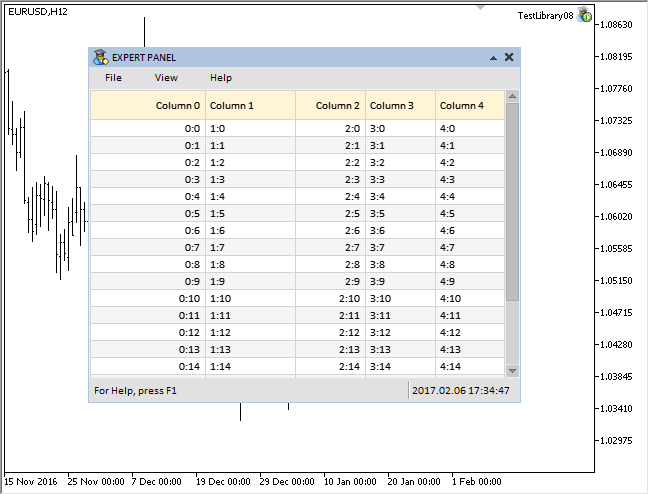### 相对于列宽调整字符串长度

```class CCanvasTable : public CElement
{private:
//- 表格数值和属性的数组   struct CTOptions
{
string            m_vrows[];
string            m_text[];      int               m_width;
ENUM_ALIGN_MODE   m_text_align;
};
CTOptions         m_vcolumns[];
};```

CCanvasTable::CorrectingText() 方法将负责调整 标题和表格单元两者内的 字符串长度。在识别操作的文本之后, 获取其宽度。接下来, 检查字符串的全文是否适合单元格, 同时考虑单元格边缘的所有偏移量。如果它适合, 将之保存在 m_text[] 数组里并离开方法。在当前版本中, 仅保存调整过的单元格文本, 但不针对标题。

1)获取字符串长度。

2)之后在循环里从最后一个字符开始遍历所有字符, 删除最后一个字符并将修剪后的文本保存在临时变量中。

4)只要有字符剩下, 获取包含省略号在内的结果字符串宽度。

5)检查依次形成的字符串是否适合表格单元, 同时考虑自单元格边缘指定的偏移。

6)如果字符串合适, 则将其保存到方法的局部变量中并停止循环。

7)此后, 将调整后的字符串保存到 m_text[] 数组中, 并从方法返回。

```class CCanvasTable : public CElement
{private:
//- 返回依据列宽调整的文本   string            CorrectingText(const int column_index,const int row_index,const bool headers=false);
};//++//| 返回依据列宽调整的文本                                               |//++string CCanvasTable::CorrectingText(const int column_index,const int row_index,const bool headers=false)
{//- 获取当前文本   string corrected_text=(headers)? m_header_text[column_index]: m_vcolumns[column_index].m_vrows[row_index];//- 自单元格边缘在 X 轴上的偏移   int x_offset=m_text_x_offset*2;//- 获取画板对象的指针   CRectCanvas *obj=(headers)? ::GetPointer(m_headers) : ::GetPointer(m_table);//- 获取文本宽度   int full_text_width=obj.TextWidth(corrected_text);//- 如果它适合单元格, 将调整后的文本保存在单独的数组中并返回   if(full_text_width<=m_vcolumns[column_index].m_width-x_offset)
{
m_vcolumns[column_index].m_text[row_index]=corrected_text;
//-      return(corrected_text);
}//- 如果文本不适合单元格, 则需要调整文本 (修剪过多字符并添加省略号)   else     {      //- 用来操纵字符串      string temp_text="";
//- 获取字符串长度      int total=::StringLen(corrected_text);
//- 从字符串中逐个删除字符, 直到所需文本宽度      for(int i=total-1; i>=0; i)
{
//- 删除一个字符         temp_text=::StringSubstr(corrected_text,0,i);
//- 如果什么都没剩下, 保留空字符串         if(temp_text=="")
{
corrected_text="";
break;
}
//- 在检查之前添加省略号         int text_width=obj.TextWidth(temp_text+"...");
//- 如果适合单元格         if(text_width<m_vcolumns[column_index].m_width-x_offset)
{
//- 保存文本并停止循环            corrected_text=temp_text+"...";
break;
}
}
m_vcolumns[column_index].m_text[row_index]=corrected_text;//- 返回调整后的文字   return(corrected_text);
}```

CCanvasTable::Text() 方法将确定是否需要调整指定列的文本, 或者发送以前调整的版本即可足矣。其代码如下所见:

```class CCanvasTable : public CElement
{private:
//- 返回文本   string            Text(const int column_index,const int row_index);
};//++//| 返回文本                                                           |//++string CCanvasTable::Text(const int column_index,const int row_index)
{
string text="";//- 如果不是更改列宽模式, 调整文本   if(m_column_resize_control==WRONG_VALUE)
text=CorrectingText(column_index,row_index);//- 如果处于更改列宽度模式下, 则...   else     {
//- ...仅宽度改变的列需要调整文本      if(column_index==m_column_resize_control)
text=CorrectingText(column_index,row_index);
//- 对于所有其它, 使用以前调整的文字      else         text=m_vcolumns[column_index].m_text[row_index];
}//- 返回文本   return(text);
}```

```class CCanvasTable : public CElement
{private:
//- 列的最小宽度   int               m_min_column_width;   //-private:
//- 更改拖拽列的宽度   void              ChangeColumnWidth(void);
};//++//| 构造器                                                            |//++CCanvasTable::CCanvasTable(void) : m_min_column_width(30)  {
...
}//++//| 更改拖拽列的宽度                                                    |//++void CCanvasTable::ChangeColumnWidth(void)
return;//- 检查标题边界的焦点   CheckColumnResizeFocus();//- 辅助变量   static int x_fixed    =0;
static int prev_width =0;//- 如果完成, 重置该值   if(m_column_resize_control==WRONG_VALUE)     {
x_fixed    =0;
prev_width =0;
return;
}//- 获取 X 轴偏移量   int xoffset=(int)m_headers.GetInteger(OBJPROP_XOFFSET);//- 得到鼠标光标的相对坐标   int x=m_mouse.X()-m_headers.X()+xoffset;//- 如果更改列宽的过程刚刚开始   if(x_fixed<1)     {
//- 保存当前列的 X 坐标和宽度      x_fixed    =x;
prev_width =m_vcolumns[m_column_resize_control].m_width;
}//- 计算列的新宽度   int new_width=prev_width+(x-x_fixed);//- 如果小于指定的极限, 保持不变   if(new_width<m_min_column_width)
return;//- 保存列的新宽度   m_vcolumns[m_column_resize_control].m_width=new_width;//- 计算表格大小   CalculateTableSize();//- 调整表的大小   ChangeTableSize();//- 表格重绘   DrawTable();
}```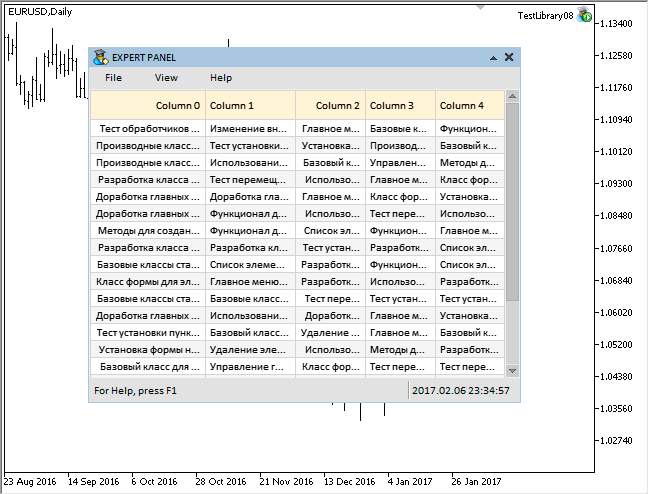### 事件处理

```//++//| 事件处理器                                                         |//++void CCanvasTable::OnEvent(const int id,const long &lparam,const double &dparam,const string &sparam)
{//- 处理光标移动事件   if(id==CHARTEVENT_MOUSE_MOVE)
{
//- 如果控件被隐藏, 离开      if(!CElementBase::IsVisible())
return;
//- 如果子窗口的数字不匹配, 离开      if(!CElementBase::CheckSubwindowNumber())
return;
//- 检查焦点覆盖在元素上      CElementBase::CheckMouseFocus();
//- 如果滚动条处于激活状态      if(m_scrollv.ScrollBarControl() || m_scrollh.ScrollBarControl())
{
ShiftTable();
return;
}
//- 更改对象颜色      ChangeObjectsColor();      //- 更改拖拽列的宽度      ChangeColumnWidth();      return;
}
...
}```

```//++//|                                                      Defines.mqh |//|                                 版权所有 2015, MetaQuotes 软件公司|//|                                              https://www.mql5.com |//++
#define ON_CHANGE_MOUSE_LEFT_BUTTON (33) // 改变鼠标左键的状态```

```//++//| 获取鼠标参数的类                                                    |//++class CMouse
{private:
//- 检查鼠标左键的状态变化   bool              CheckChangeLeftButtonState(const string mouse_state);
};//++//| 处理移动鼠标光标的事件                                               |//++void CMouse::OnEvent(const int id,const long &lparam,const double &dparam,const string &sparam)
{//- 处理光标移动事件   if(id==CHARTEVENT_MOUSE_MOVE)
{
//- 坐标和鼠标左键的状态      m_x                 =(int)lparam;
m_y                 =(int)dparam;
m_left_button_state =CheckChangeLeftButtonState(sparam);      ...
}
}//++//| 检查鼠标左键的状态变化                                               |//++bool CMouse::CheckChangeLeftButtonState(const string mouse_state)
{
bool left_button_state=(bool)int(mouse_state);//- 发送关于鼠标左键状态更改的消息   if(m_left_button_state!=left_button_state)
::EventChartCustom(m_chart.ChartId(),ON_CHANGE_MOUSE_LEFT_BUTTON,0,0.0,"");//-   return(left_button_state);
}```

•  将类中的某些字段归零;

•  调整滚动条;

•  重绘表格

```//++//| 事件处理器                                                         |//++void CCanvasTable::OnEvent(const int id,const long &lparam,const double &dparam,const string &sparam)
{//- 更改鼠标左键的状态   if(id==CHARTEVENT_CUSTOM+ON_CHANGE_MOUSE_LEFT_BUTTON)
{
return;
//- 如果鼠标左键释放      if(!m_mouse.LeftButtonState())
{
//- 重置宽度更改模式         m_column_resize_control=WRONG_VALUE;
//- 隐藏光标         m_column_resize.Hide();
//- 考虑最近变化来调整滚动条         HorizontalScrolling(m_scrollh.CurrentPos());        }
//- 更改对象颜色      ChangeObjectsColor();     }
}```

### 结论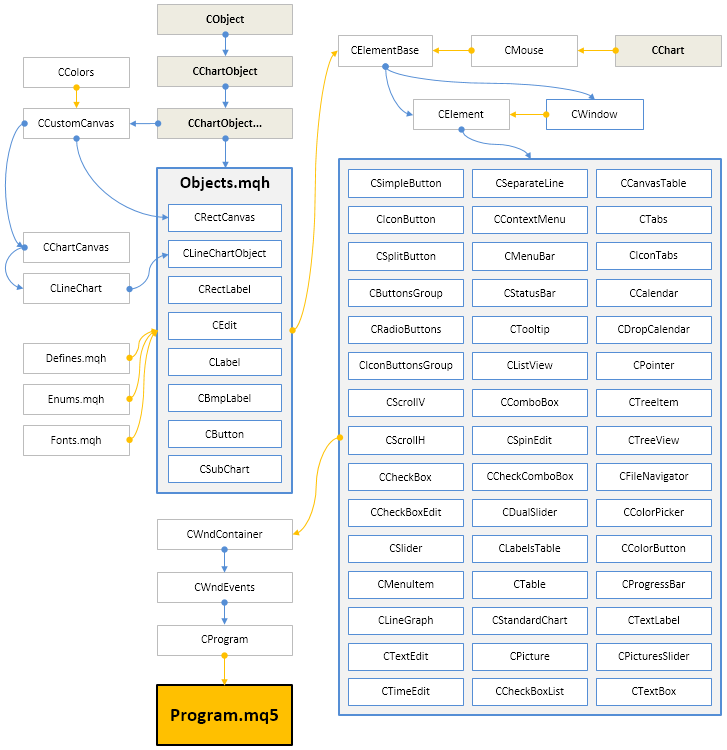## 量化课程

移动端课程